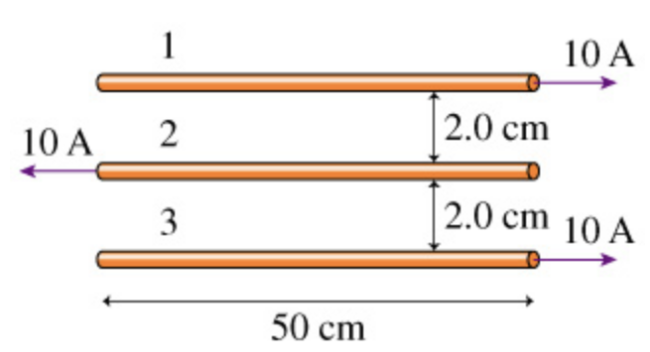# Problem: a. What is the magnitude of the net force on the first wire in (Figure 1)?b. What is the magnitude of the net force on the second wire in (Figure 1)?c. What is the magnitude of the net force on the third wire in (Figure 1)?

###### FREE Expert Solution

The magnitude of magnetic force between parallel current-carrying wires is:

$\overline{){\mathbf{F}}{\mathbf{=}}\frac{{\mathbf{\mu }}_{\mathbf{0}}{\mathbf{i}}_{\mathbf{1}}{\mathbf{i}}_{\mathbf{2}}\mathbf{l}}{\mathbf{2}\mathbf{\pi r}}}$

a.

F1 = F12 - F13

$\begin{array}{rcl}{\mathbf{F}}_{\mathbf{1}}& \mathbf{=}& \frac{{\mathbf{\mu }}_{\mathbf{0}}{\mathbf{i}}_{\mathbf{1}}{\mathbf{i}}_{\mathbf{2}}\mathbf{l}}{\mathbf{2}{\mathbf{\pi r}}_{\mathbf{1}}}\mathbf{-}\frac{{\mathbf{\mu }}_{\mathbf{0}}{\mathbf{i}}_{\mathbf{1}}{\mathbf{i}}_{\mathbf{3}}\mathbf{l}}{\mathbf{2}{\mathbf{\pi r}}_{\mathbf{2}}}\\ & \mathbf{=}& \frac{\mathbf{\left(}\mathbf{4}\mathbf{\pi }\mathbf{×}{\mathbf{10}}^{\mathbf{-}\mathbf{7}}\mathbf{\right)}\mathbf{\left(}\mathbf{10}\mathbf{\right)}\mathbf{\left(}\mathbf{10}\mathbf{\right)}\mathbf{\left(}\mathbf{50}\mathbf{×}{\mathbf{10}}^{\mathbf{-}\mathbf{2}}\mathbf{\right)}}{\mathbf{2}\mathbf{\pi }\mathbf{\left(}\mathbf{2}\mathbf{.}\mathbf{0}\mathbf{×}{\mathbf{10}}^{\mathbf{-}\mathbf{2}}\mathbf{\right)}}\mathbf{-}\frac{\mathbf{\left(}\mathbf{4}\mathbf{\pi }\mathbf{×}{\mathbf{10}}^{\mathbf{-}\mathbf{7}}\mathbf{\right)}\mathbf{\left(}\mathbf{10}\mathbf{\right)}\mathbf{\left(}\mathbf{10}\mathbf{\right)}\mathbf{\left(}\mathbf{50}\mathbf{×}{\mathbf{10}}^{\mathbf{-}\mathbf{2}}\mathbf{\right)}}{\mathbf{2}\mathbf{\pi }\mathbf{\left(}\mathbf{4}\mathbf{.}\mathbf{0}\mathbf{×}{\mathbf{10}}^{\mathbf{-}\mathbf{2}}\mathbf{\right)}}\end{array}$

100% (198 ratings)###### Problem Detailsa. What is the magnitude of the net force on the first wire in (Figure 1)?

b. What is the magnitude of the net force on the second wire in (Figure 1)?

c. What is the magnitude of the net force on the third wire in (Figure 1)?